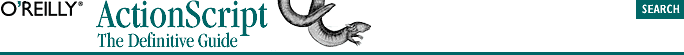home | O'Reilly's CD bookshelfs | FreeBSD | Linux | Cisco | Cisco Exam## 5.8. The Grouping Operator

`(expression)`

The expression within the parentheses is evaluated and returned. Here are some examples:

```if (x == y) {                 // Syntactically required for if statement
trace("x and y are equal"); // Required for the function call
}
(5 + 6) * 7                   // Force a nonstandard order of operation
(x >= 100) || (y <= 50)       // Parentheses not required but added for legibility
x >= 100 || y <= 50           // No parentheses...a little harder to read```